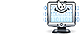Unit Conversion Software Web Widgets Loan Calculator Currency Rates Country Flags Unit Converter
 Faq HelpWatt/Square Meter ConversionContact

Watt/Square Meter, unit of measure

To perform conversions between watt/square meter and other Heat Flux Density units please try our Heat Flux Density Unit Converter

Convert watt/square meter to:
kilowatt/square meter, watt/square centimeter, watt/square inch, joule/second/square meter, kilocalorie (IT)/hour/square meter, kilocalorie (IT)/hour/square foot, calorie (IT)/second/square centimeter, calorie (IT)/minute/square centimeter, calorie (IT)/hour/square centimeter, calorie (th)/second/square centimeter, calorie (th)/minute/square centimeter, calorie (th)/hour/square centimeter, dyne/hour/centimeter, erg/hour/square millimeter, foot pound/minute/square foot, horsepower/square foot, horsepower (metric)/square foot, Btu (IT)/second/square foot, Btu (IT)/minute/square foot, Btu (IT)/hour/square foot, Btu (th)/second/square inch, Btu (th)/second/square foot, Btu (th)/minute/square foot, Btu (th)/hour/square foot, CHU/hour/square footDownload Heat Flux Density Unit Converter
our powerful software utility that helps you make easy conversion between more than 2,100 various units of measure in more than 70 categories. Discover a universal assistant for all of your unit conversion needs - download the free demo version right away!Instantly add a free Heat Flux Density Converter Widget to your website
It will take less than a minute, is as easy as cutting and pasting. The converter will blend seamlessly into your website since it is fully rebrandable.Looking for an interactive heat flux density conversion table? Visit our forum to discuss conversion issues and ask for free help! Try the instant categories & units searchit gives you results as you type!Copyright © 1998-2009 UnitConversion.org Privacy & Terms | About | Faq | Help | Contact | Link to Us